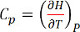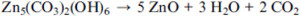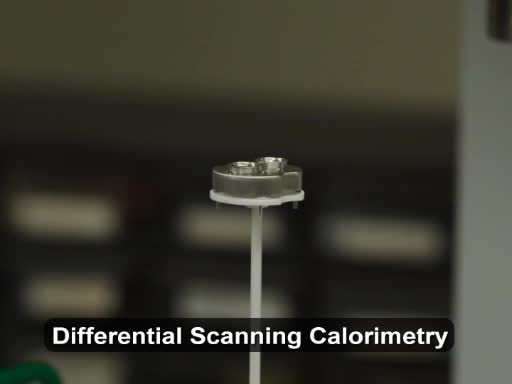Trial ends in

# Using Differential Scanning Calorimetry to Measure Changes in Enthalpy

### Overview

Source: Laboratory of Dr. Terry Tritt — Clemson University

Differential Scanning Calorimetry (DSC) is a method of thermodynamic analysis based on heat-flux method, wherein a sample material (enclosed in a pan) and an empty reference pan are subjected to identical temperature conditions. The energy difference that is required to maintain both the pans at the same temperature, owing to the difference in the heat capacities of the sample and the reference pan, is recorded as a function of temperature. This energy released or absorbed is a measure of the enthalpy change (ΔΗ) of the sample with respect to the reference pan.

### Principles

The DSC can be used to measure the heat capacity of material systems, as well as the change of enthalpy (ΔΗ) for dramatic phase transformation processes, chemical reactions, ionizations, dissolutions in solvents, vacancy formation, and so on. The standard enthalpy of formation is defined as the change in enthalpy, when one mole of a substance in the standard state are formed from elemental constituents in their stable states.1

The DSC measurement setup consists of a furnace and an integrated sensor connected to thermocouples with designated positions for the sample and the reference pans. The temperature of the sample and the reference are controlled independently using separate but identical ovens. The DSC measurement is carried out in three steps: baseline measurement using empty pan and reference, standard reference measurement to test accuracy, and the sample measurement.

This video explains the sample preparation and the technique of measurement of enthalpy of formation of an oxide via decomposition of a carbonate.

### Procedure

1. Baseline Measurement

1. Controller, Measuring unit, Computer system, Thermostat approximately 60 min. before starting the measurement. Purge gases must be connected to the system.
2. Place two empty crucibles (with lid) into the sample carrier. The crucible material may be chosen based on the temperature range to be measured.
3. Move the furnace to measuring position.
4. Adjust the measuring conditions (gas, vacuum).
5. Start the measurement program.
6. Proceed to create a baseline measurement using Sample Mass = 0.
7. Open Temperature Recalibration, Open Sensitivity programs.
8. Set Temperature Program, initial temperature, heating rate.
9. Set the initial conditions and the temperature threshold values. After purging the system with Argon/nitrogen gas a few times, allow the gas to continuously flow through the system, adjusting the flow rate to a steady rate (e.g. 50 mL/min).
10. Start the measurement.
11. The DSC measurements are started at room temperature after an initial stabilization at the starting temperature. The temperature stabilization is important step to avoid any offset due to a difference in the thermal capacities of the sample pan and the reference pan and contents. A steady heating rate of 20 °C /min, under Argon gas atmosphere is generally used. The range of temperature is determined according to the sample and the temperature range of interest.

2. Standard Sample Measurement to Ensure Accuracy of the System

1. Open the measuring unit after the furnace has cooled down.
2. Remove the empty crucible that is designated as the sample pan.
3. Choose the standard depending on the temperature range to be measured.
4. Weigh the standard. A finely polished synthetic sapphire (carborundum, aluminium oxide) disk is used as heat capacity and transformation enthalpy standard. Sapphire is stable over a wide range of temperature, and its heat capacity has been accurately determined over a wide range of temperature.
5. Insert standard sample carefully in the sample crucible using tweezers.
6. Move the furnace to measuring position.
7. Adjust the measuring conditions (gas, vacuum).
8. Proceed as follows to combine the standard measurement with the correction measurement:
9. Use sample mass = x mg (mass of standard sample).
10. Open Temperature Recalibration, Open sensitivity
11. Use the same Temperature program (temperature program remains the same as the baseline temperature program)
12. Start the measurement.
13. Set the initial conditions and the temperature threshold values. After purging the system a few times, allow the purging gas to continuously flow through the system, adjusting the flow rate.
14. Measurement conditions (e.g. heating rate, gases, type of crucible) for the baseline and the subsequent standard measurement must be the same.
15. Using the same sensitivity and temperature calibration files, the start program to measure the standard sample.

3. Sample Measurement

1. Polish the sample surfaces. Place the flattest sample surface facing the bottom of the pan. Use an optimal sample size that fits the pan, without touching the lid. The sample is finely polished to obtain good thermal contact with the sample pan, so the temperature can be accurately determined and the data is less noisy.
2. Measure the sample mass accurately.
3. Open the measuring unit after the furnace has cooled down.
4. Remove the standard sample from the crucible.
5. Clean the crucible using alcohol. Insert the sample to be measured in the crucible replacing the standard.
6. Follow step 3 to measure the sample. The measurement conditions (e.g. heating rate, gases, type of crucible) for the baseline measurement and the subsequent standard and sample measurement must be the same.
7. Follow step 3 to complete the measurement.

Energy changes that occur during chemical reactions are defined by the term enthalpy, and are an important concept in thermodynamics. While enthalpy itself cannot be measured, the change in enthalpy in a system can be, and accounts for the energy transferred between a system and its environment during a chemical process at constant pressure.

Chemical reactions that give off energy to their surroundings, primarily as heat, are described as exothermic and have a negative enthalpy change. Some rapid exothermic reactions give off so much heat that they are explosive. In other reactions, energy is absorbed from the environment. These reactions are endothermic and have a positive enthalpy change. It is important to understand the enthalpy change in a chemical reaction, so that the reaction can be performed safely and efficiently. Enthalpy change can be measured experimentally using differential scanning calorimetry, or DSC. DSC is a thermodynamic analysis method based on the concept of heat flow. This video will demonstrate how to use Differential Scanning Calorimetry to measure the enthalpy of reaction of an oxide via the decomposition of a carbonate.

Enthalpy is a state function, meaning that it only depends on the initial and final states of a reaction and is path independent. Elevation is an example of a state function, as it depends only on the height difference between the base and peak. The hiker and climber take different routes to the top. No matter which path they use to reach the top, they both travel the same overall elevation. A similar concept is applied to thermodynamics, where the change in enthalpy between the beginning and end of the reaction is used to understand energy changes during the reaction.

Hess's Law defines enthalpy for a chemical reaction, denoted as ΔH, as the sum of the enthalpies of each reaction product minus the sum of the enthalpies of reactants. Enthalpies of common substances are published and readily available. These published values can be used to calculate enthalpy change in common reactions. This example shows the enthalpy calculation for the formation of nitrogen dioxide gas from nitric oxide and oxygen. The enthalpy values of each component can be found in the chart and substituted into the equation. "n" accounts for the number of moles of each component, and must be included in the calculation. This reaction has a negative enthalpy, meaning it is exothermic.

Enthalpy change can also be measured experimentally using DSC. The DSC measurement setup consists of separate sample and reference pans attached to temperature sensors. The temperature of the sample pan, containing the compound of interest, and the reference pan, which typically remains empty, are controlled independently using separate but identical heaters.

The temperature of both pans is increased linearly. The difference in the amount of energy, or heat flow, required to maintain both pans at a constant temperature is recorded as a function of temperature. For example, if the sample pan contains a material that absorbs energy when it undergoes a phase change or reaction, the heater under the sample pan must apply more energy to increase the pan temperature than the heater under the empty reference pan. This difference in heat flow is directly proportional to enthalpy. Now that you have learned the basics of enthalpy, let's see how to run the enthalpy measurement.

To begin the DSC measurement, switch the instrument on by powering on the controller, measuring unit, computer system, and cooling water. First, a baseline measurement is made by running the DSC with empty reference and sample pans. The baseline will be used to normalize the sample measurements later on.

Chose a pan that is chemically inert, and stable in the desired temperature range. At temperatures higher than 600 degrees, platinum/rhodium pans with aluminum oxide liners are commonly used. Place the empty sample and reference pans with lids into the sample holder.

Check that inert gas lines are connected to the system. Purge the system, and adjust the flow to a steady state.

Set the baseline parameters using a sample mass of zero. Input the temperature range and the heating rate. Allow the system to stabilize at 40 °C for 10 min in order to avoid offsets caused by differences in the thermal properties of the sample and reference pans. With the system stabilized, the baseline can be measured.

Next, a reference measurement is performed using a standard sample to test the accuracy of the instrument. Open the measuring unit after the furnace has cooled to room temperature, and remove the empty sample pan. Leave the reference pan in the instrument.

Select a standard sample with known thermodynamic properties in the desired temperature range, in order to test the instrument accuracy. A finely polished synthetic sapphire disk is used as the standard because its thermal properties are well reported over a wide range of temperatures.

Weigh the standard sample with a high precision balance. Carefully insert the standard into the sample pan using tweezers. Be sure to use the same pan used in the baseline measurement. Insert the pan into the instrument, and close the sample chamber. Allow the purge gas flow to stabilize, and the standard to stabilize to room temperature. Input the mass of the standard sample and set the heating program, using the same temperature parameters used for the baseline measurement. Then begin the measurement.

The plot of this standard sample can be used to assess the accuracy of the instrument.

Now that the baseline and standard measurements have been made, the sample can be measured. Open the measuring unit after the furnace has fully cooled, and remove the reference sample from the pan. Clean the pan thoroughly with alcohol, as it will be used for the sample measurement. Add a small amount of sample to the pan. For powdered solids, as with the calcium carbonate in this example, ensure that the sample powder is evenly distributed at the bottom of the pan.

Next, weigh the sample and pan. The mass should be similar to the standard sample for accuracy. Run the sample measurement using the accurate sample weight and identical heating parameters to both the baseline and standard measurements.

DSC data is presented as a plot of heat flow, or q, versus temperature, also called a thermoanalytical curve. Endothermic events appear as positive features, whereas exothermic events appear as negative features.

Dividing heat flow by the heating rate gives the heat capacity. Heat capacity, or Cp, is defined as the amount of energy required to raise the temperature of a substance by one degree Celsius. Assuming constant pressure, the change in enthalpy per degree is equivalent to the heat capacity of a material. Thus, the enthalpy change is obtained by calculating the area under the curve between two temperature limits. In this example, the enthalpy of decomposition of calcium carbonate to form calcium oxide, or quicklime, is analyzed with the DSC. This process is commonly known as calcination. The decomposition of calcium carbonate occurs endothermically, as evidenced by the positive peak at 853 degrees Celsius. The enthalpy of decomposition of calcium carbonate is calculated from the area under the peak and is approximately 160 kilojoules per mole. The calculated value via Hess's Law was 178 kilojoules per mole. Discrepancies between the measured and calculated values may arise from non-ideal conditions and measurement artifacts.

Enthalpy is an important concept in describing energy flow in many different systems, aside from chemical reactions. Enthalpy can also be used to understand phase transformations in materials and mixtures.

Polymers are materials used in a wide range of applications. In this example, porous copolymer structures of polystyrene, PS, and polyvinyl pyridine, P4VP, were analyzed.

Enthalpy change occurred during the phase transition in each polymer component and was visualized using DSC. The glass transition temperature, or Tg, describes the point at which an amorphous material transitions from a rigid glassy state to a viscous fluid state, and appears as a ridge in the scan.

The melting temperature describes the point at which a rigid crystalline material transitions to a viscous fluid state, and is visualized as an endothermic peak. The melting temperature for one polymer component was visualized in this example.

DSC can also be used to analyze phase transitions in biological samples. In this example, the phase transition of a cell suspension was analyzed in order to understand its freeze-drying properties. Freeze-drying, or lyophilization, is commonly used for long-term storage of biological samples. Here, cell suspensions were prepared and frozen under different conditions in the DSC instrument. The frozen suspensions were then heated, and the Tg measured. Later, the cells were analyzed with electron microscopy to determine which freezing condition promoted cell survival. An understanding of the freeze drying process via phase transition temperatures helps tailor the process in order to help improve cell storage. Enthalpy is also used to study miscibility, or the ability of a mixture to form a homogeneous solution. In this example, mixtures of protein were analyzed with DSC in order to examine the miscibility of the different mixtures. An immiscible mixture may exhibit several transition features in a DSC scan, as each component will undergo a phase transition separately. Whereas a homogenous mixture exhibits one phase transition feature.

You have just watched JoVE's introduction to Enthalpy using Differential Scanning Calorimetry. You should now understand the theory of enthalpy and how to use DSC to measure it.

Thanks for watching!

### Results

ZnO formation via Decomposition of ZnCO3

The change in enthalpy per degree, at constant pressure is equivalent to the heat capacity of a material at constant pressure given by Equation 1. The enthalpy change is obtained by estimating the area under the curve between two temperature limits given by Equation 2.(Equation 1)(Equation 2)

Using specific software, the area under the curve is obtained from any heat capacity measurement. The DSC provides a comparative accurate method of measuring heat capacities and enthalpy changes.

A representative result of the decomposition of zinc carbonate (ZnCO3) forming ZnO is shown below. By the process of calcination, ZnCO3 decomposes to ZnO releasing carbon dioxide. Using a starting composition of Zn5(CO3)2(OH)6 a broad exothermic peak around 281 °C was reported by Liu et al.2 following the release of H2O and CO2 according to Equation 3.(Equation 3)

The enthalpy of transformation of Zn5(CO3)2(OH)6 to ZnO may be estimated by calculating the area under the curve, at the point of decomposition given by the following exothermic peak. Using Hess’s law of constant heat summation, the enthalpy of formation of ZnO may be estimated.

### Applications and Summary

A major application area of DSC is the glass transition (Tg) in amorphous polymers, in which the material changes from a rigid glassy state to a viscous liquid state. Pharmaceutical research on nano-particles is also an emerging field, where the DSC has been used to quantify amorphous or crystalline phase in nano-solids. A review of DSC techniques on applications in biology and nano-science has been provided by Gill et al.3 Nanostructured lipid carriers (NLC) have potential applications in medicine and have been considered as drug delivery carriers.

Calorimetry is a method of analyzing thermal properties of materials to determine the enthalpy change associated with a physical or chemical reaction of interest. Calorimeters are frequently used for quantifying amorphous or crystalline phases. More recently, DSC measurements are used in the fields of nano-science and biochemistry to measure thermodynamic properties of nano-sized bio-molecules. The DSC can also be used to analyze the chemical changes in an oxidized sample. The enthalpy of formation of different metal oxides is useful for metallurgical and industrial calculations.

The estimation of heat of formation of oxides generally requires the combustion of the specific metal in oxygen inside a calorimeter, which may lead to damage of expensive sensors and thermocouples of the particular equipment. The estimation of heat of formation of an oxide, via calcination process through the decomposition of a carbonate producing non-toxic carbon-dioxide gas, gives a simpler method of estimation of the heat of formation of the corresponding oxide. The estimation of the enthalpy of transformation of carbonates is not only applicable for modeling of geochemical process, but also useful for fundamental research, and industrial applications.

### Disclosures

No conflicts of interest declared.Play Video

### Cite this

JoVE Science Education Database. General Chemistry. Using Differential Scanning Calorimetry to Measure Changes in Enthalpy. JoVE, Cambridge, MA, (2021).More

JoVE Science Education Database. General Chemistry. Using Differential Scanning Calorimetry to Measure Changes in Enthalpy. JoVE, Cambridge, MA, (2021).

Less
Copy Citation
View Video

### Get cutting-edge science videos from JoVE sent straight to your inbox every month.X